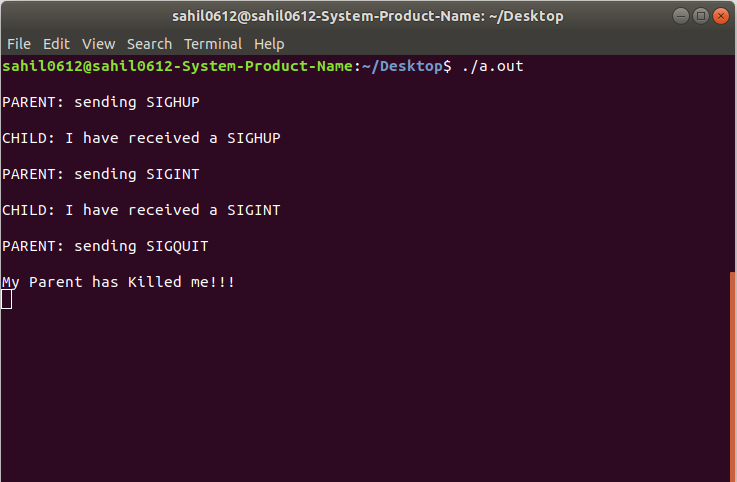Open in App
Not now

# Communication between two process using signals in C

• Difficulty Level : Easy
• Last Updated : 31 Jan, 2019

Prerequisite : C signal handling

In this post, the communication between child and parent processes is done using kill() and signal(), fork() system call.

• fork() creates the child process from the parent. The pid can be checked to decide whether it is the child (if pid == 0) or the parent (pid = child process id).
• The parent can then send messages to child using the pid and kill().
• The child picks up these signals with signal() and calls appropriate functions.

Example of how 2 processes can talk to each other using kill() and signal():

 `// C program to implement sighup(), sigint() ` `// and sigquit() signal functions ` `#include ` `#include ` `#include ` `#include ` `#include ` ` `  `// function declaration ` `void` `sighup(); ` `void` `sigint(); ` `void` `sigquit(); ` ` `  `// driver code ` `void` `main() ` `{ ` `    ``int` `pid; ` ` `  `    ``/* get child process */` `    ``if` `((pid = fork()) < 0) { ` `        ``perror``(``"fork"``); ` `        ``exit``(1); ` `    ``} ` ` `  `    ``if` `(pid == 0) { ``/* child */` `        ``signal``(SIGHUP, sighup); ` `        ``signal``(SIGINT, sigint); ` `        ``signal``(SIGQUIT, sigquit); ` `        ``for` `(;;) ` `            ``; ``/* loop for ever */` `    ``} ` ` `  `    ``else` `/* parent */` `    ``{ ``/* pid hold id of child */` `        ``printf``(``"\nPARENT: sending SIGHUP\n\n"``); ` `        ``kill(pid, SIGHUP); ` ` `  `        ``sleep(3); ``/* pause for 3 secs */` `        ``printf``(``"\nPARENT: sending SIGINT\n\n"``); ` `        ``kill(pid, SIGINT); ` ` `  `        ``sleep(3); ``/* pause for 3 secs */` `        ``printf``(``"\nPARENT: sending SIGQUIT\n\n"``); ` `        ``kill(pid, SIGQUIT); ` `        ``sleep(3); ` `    ``} ` `} ` ` `  `// sighup() function definition ` `void` `sighup() ` ` `  `{ ` `    ``signal``(SIGHUP, sighup); ``/* reset signal */` `    ``printf``(``"CHILD: I have received a SIGHUP\n"``); ` `} ` ` `  `// sigint() function definition ` `void` `sigint() ` ` `  `{ ` `    ``signal``(SIGINT, sigint); ``/* reset signal */` `    ``printf``(``"CHILD: I have received a SIGINT\n"``); ` `} ` ` `  `// sigquit() function definition ` `void` `sigquit() ` `{ ` `    ``printf``(``"My DADDY has Killed me!!!\n"``); ` `    ``exit``(0); ` `} `

Output:My Personal Notes arrow_drop_up
Related Articles